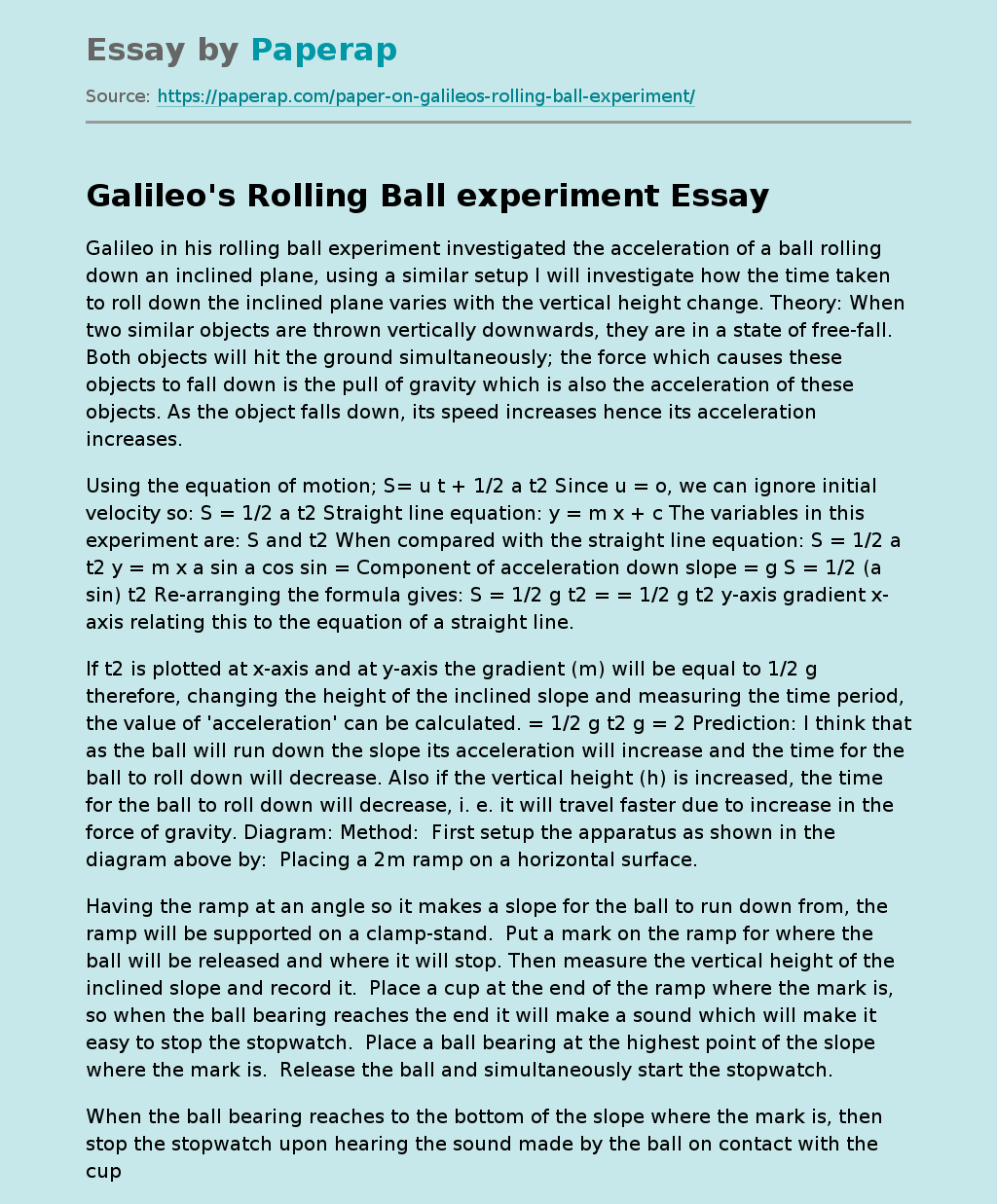# Galileo's Rolling Ball experiment

Galileo in his rolling ball experiment investigated the acceleration of a ball rolling down an inclined plane, using a similar setup I will investigate how the time taken to roll down the inclined plane varies with the vertical height change. Theory: When two similar objects are thrown vertically downwards, they are in a state of free-fall. Both objects will hit the ground simultaneously; the force which causes these objects to fall down is the pull of gravity which is also the acceleration of these objects.

As the object falls down, its speed increases hence its acceleration increases.

Using the equation of motion; S= u t + 1/2 a t2 Since u = o, we can ignore initial velocity so: S = 1/2 a t2 Straight line equation: y = m x + c The variables in this experiment are: S and t2 When compared with the straight line equation: S = 1/2 a t2 y = m x a sin a cos sin = Component of acceleration down slope = g S = 1/2 (a sin) t2 Re-arranging the formula gives: S = 1/2 g t2 = = 1/2 g t2 y-axis gradient x-axis relating this to the equation of a straight line.

If t2 is plotted at x-axis and at y-axis the gradient (m) will be equal to 1/2 g therefore, changing the height of the inclined slope and measuring the time period, the value of ‘acceleration’ can be calculated. = 1/2 g t2 g = 2 Prediction: I think that as the ball will run down the slope its acceleration will increase and the time for the ball to roll down will decrease.

Get quality help nowMarrie pro writerVerified

Proficient in: Force5 (204)

“ She followed all my directions. It was really easy to contact her and respond very fast as well. ”+84 relevant experts are online

Also if the vertical height (h) is increased, the time for the ball to roll down will decrease, i. e. it will travel faster due to increase in the force of gravity. Diagram: Method:  First setup the apparatus as shown in the diagram above by:  Placing a 2m ramp on a horizontal surface.

Having the ramp at an angle so it makes a slope for the ball to run down from, the ramp will be supported on a clamp-stand.  Put a mark on the ramp for where the ball will be released and where it will stop. Then measure the vertical height of the inclined slope and record it.  Place a cup at the end of the ramp where the mark is, so when the ball bearing reaches the end it will make a sound which will make it easy to stop the stopwatch.  Place a ball bearing at the highest point of the slope where the mark is.  Release the ball and simultaneously start the stopwatch.

When the ball bearing reaches to the bottom of the slope where the mark is, then stop the stopwatch upon hearing the sound made by the ball on contact with the cup.  Repeat the experiment several times and get an average for all repeats to get a more accurate result. Safety: * When carrying out the experiment make sure the ramp is securely held on the clamp. * Handle the ramp carefully when carrying it around. Do not swing it around. * When changing the height, first remove the ramp then adjust the height before returning the ramp to its place. Vertical Height (h) in metres Time (s) Average time.

Time squared (t2) 0. 10 10. 00 3. 88 4. 06 4. 06 4. 00 16. 00 0. 20 5. 00 3. 21 2. 89 2. 93 2. 99 8. 94 0. 30 3. 33 2. 38 2. 22 2. 38 2. 33 5. 43 0. 40 2. 50 1. 95 1. 99 2. 02 1. 99 3. 96 0. 50 2. 00 1. 89 1. 76 1. 82 1. 80 3. 24 0. 60 1. 67 1. 65 1. 66 1. 66 1. 66 2. 76 Results table: Graph: Evaluation: Although I carried out the experiment as accurately as I could there wree still many sources of error in the method I used. Firstly the error in my timing has been quoted as + 0. 2 s. this is because of my reaction time i. e. the time for the signal from the brain to be transferred to the hand.

There is an error in both the starting and stopping of the stopwatch, which makes this the most significant source of error in this experiment. In order to improve the experiment the timing error needs to be reduced. This can be done by increasing the number of readings for every height. Increasing the number from 3 readings to 6 readings would half the percentage error in the results as shown below: Max % error (with 3 readings) = = 6. 7% Max % error (with 6 readings) = = 3. 3% From the above calculation it can be clearly seen that increasing the number of readings significantly reduces the % error by 3.4% or by half.

Another way of increasing the accuracy of the timing would be to use a motion sensor to record the time. The maximum percentage error in the height measurement is: = 10% This error could be reduced by measuring the height of the clamp accurately using a ruler with a mm scale. The percentage error in the experiment was: Error in height + (2x error in time) = 10 + 6. 7 = 16. 7% From looking at the graph the points are scattered further away from the line of best fit as the height was increased. This is expected because the percentage error is greatest at these values.